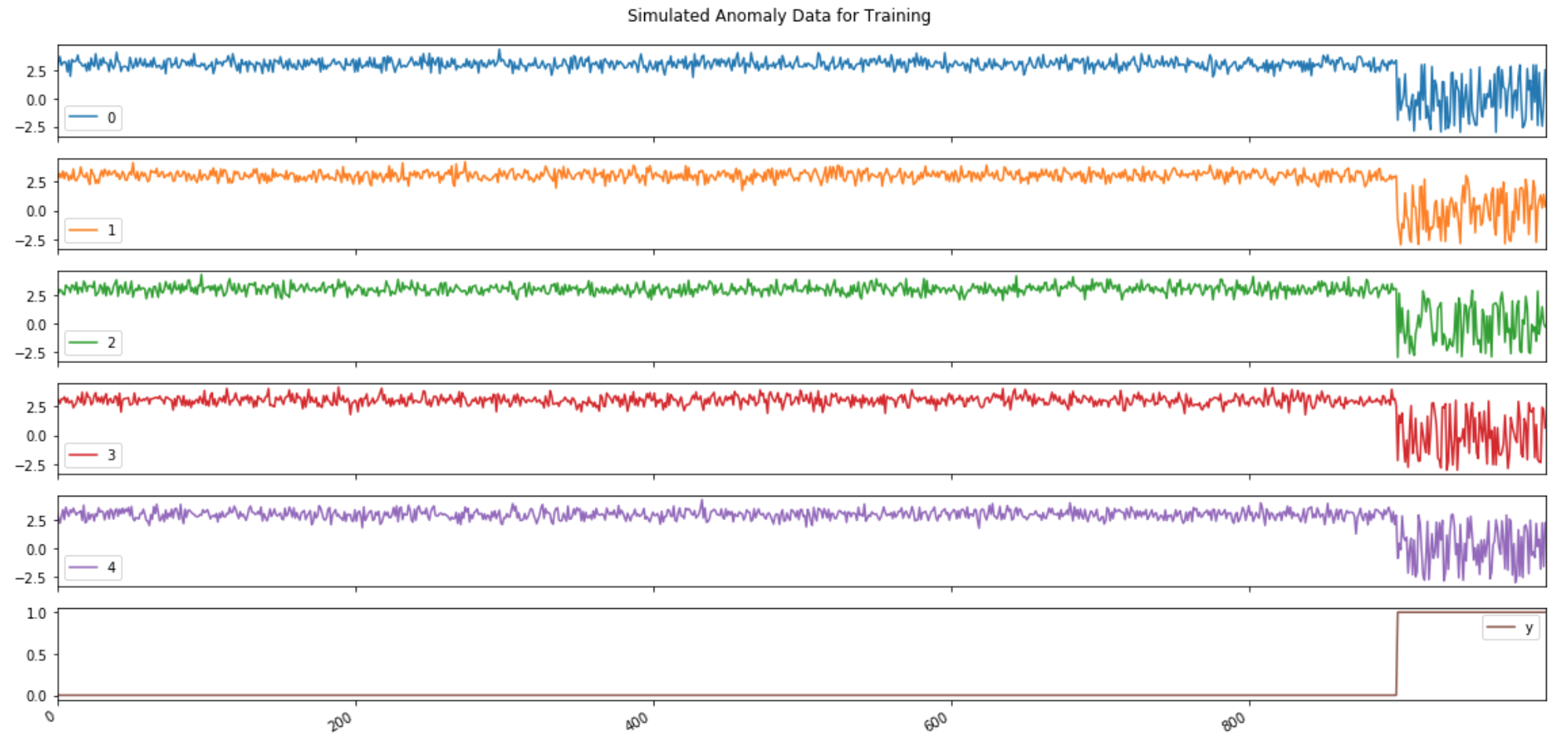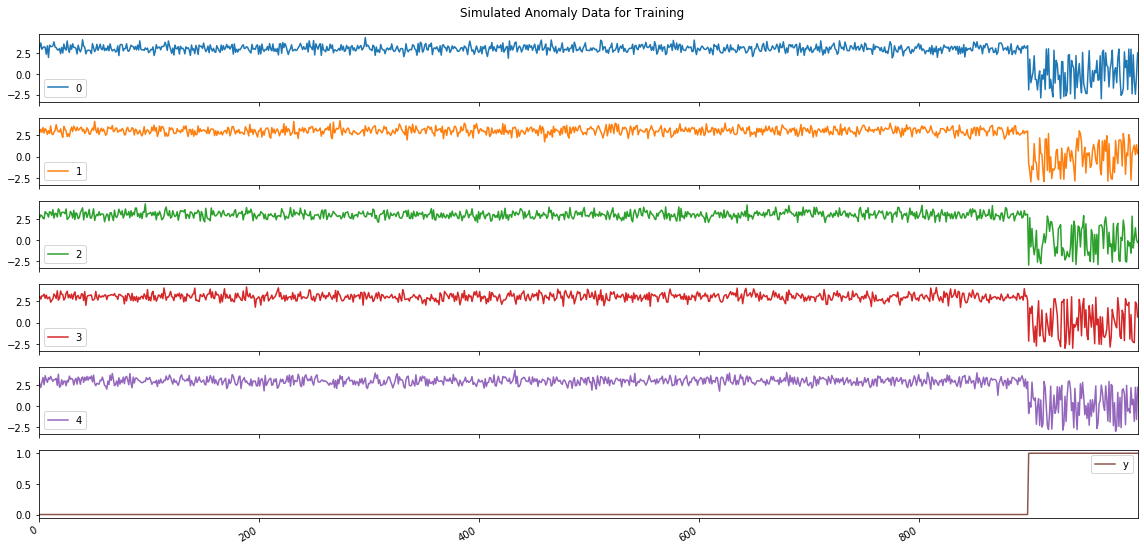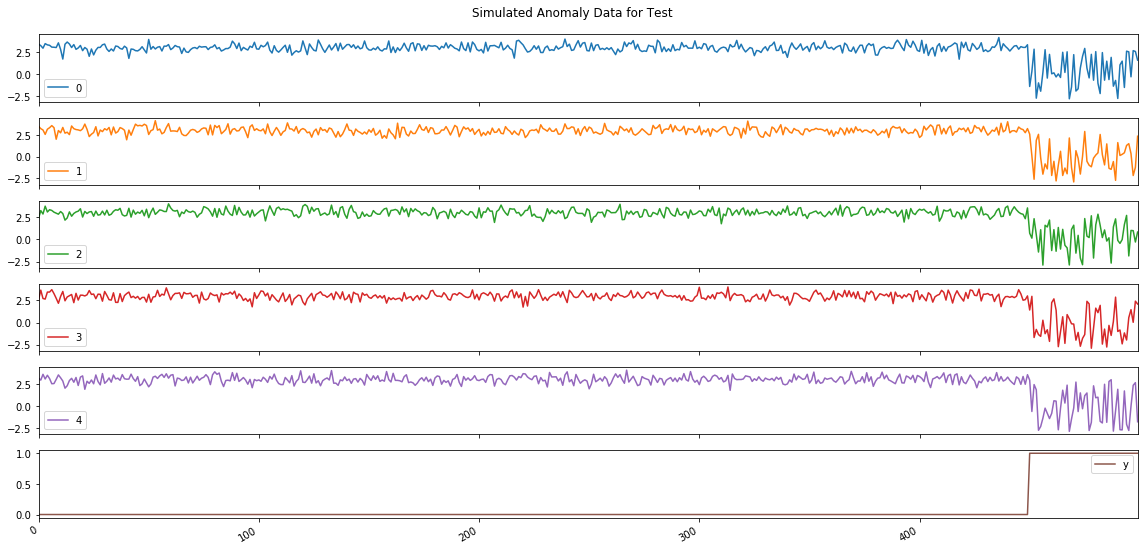# Make Simulated Data For Anomaly Detection

## Goal¶

This post aims to introduce how to make simulated data for anomaly detection using PyOD, which is outlier detection package.Reference

## Libraries¶

In :
import pandas as pd
import numpy as np
import matplotlib.pyplot as plt
%matplotlib inline

# PyOD
from pyod.utils.data import generate_data, get_outliers_inliers


## Create an anomaly dataset¶

### Create random data with 5 features¶

In :
X_train, X_test, y_train, y_test = generate_data(behaviour='new', n_features=5)
df_tr = pd.DataFrame(X_train)
df_tr['y'] = y_train
df_te = pd.DataFrame(X_test)
df_te['y'] = y_test

In :
df_tr.head()

Out:
0 1 2 3 4 y
0 2.392715 3.084379 2.972580 2.907177 3.155727 0.0
1 3.185049 2.789920 2.648234 3.062398 2.673828 0.0
2 3.683184 3.169288 2.973224 2.725969 2.213359 0.0
3 2.928545 2.823802 2.888037 3.109228 2.813928 0.0
4 3.112898 3.365741 2.599102 3.090721 3.391458 0.0

### Visualize created anomaly data¶

In :
axes = df_tr.plot(subplots=True, figsize=(16, 8), title='Simulated Anomaly Data for Training');
plt.tight_layout(rect=[0, 0.03, 1, 0.95])In :
axes = df_te.plot(subplots=True, figsize=(16, 8), title='Simulated Anomaly Data for Test');
plt.tight_layout(rect=[0, 0.03, 1, 0.95])